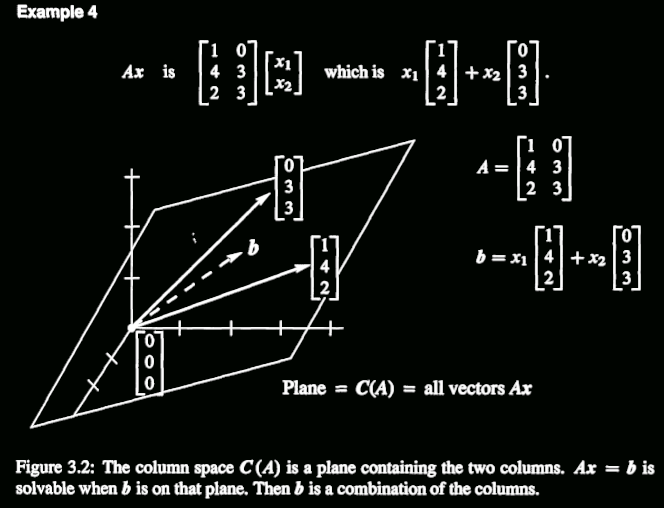Abstract: 本章介绍线性代数的核心内容，关于Vectors Space和subspace的一些观点，本文作为第一篇，主要说明基础知识
Keywords: 向量空间，子空间，列空间，张成

## 向量空间（Spaces of Vectors）

$$v=\begin{bmatrix}1\\2\end{bmatrix}$$

$$v=\begin{bmatrix}x_1\\x_2\end{bmatrix}\\ x_1,x_2 \in \Re$$

$$\Re^2$$

$$u=v=\begin{bmatrix}book\\food\\number\end{bmatrix}\\$$

### $R^n$

$$v=\begin{bmatrix}x_1\\x_2\ \vdots\\x_n\end{bmatrix}\\ x_1,x_2, \dots ,x_n \in \Re$$

$$\Re^n$$

### $C^n$

$$v=\begin{bmatrix}c_1\\c_2\ \vdots\\c_n\end{bmatrix}\\ c_1,c_2, \dots ,c_n \in C$$

$$C^n$$

### M,F and Z

M:The vector space of all real 2x2 matrices
F:The vector spcae of all real functions f(x)
Z:The vector space that consists only of a zero vector

$$a_0+a_1x+a_2x^2+\dots+a_nx^n$$
Z向量空间值得说一下，我们平时可以把它写成
$$\Re^0$$

## 8 Conditions（八项基本原则）

1、要同党中央保持高度一致，不阳奉阴违、自行其是；
2、要遵守民主集中制，不独断专行、软弱放任；
3、要依法行使权力，不滥用职权、玩忽职守；
4、要廉洁奉公，不接受任何影响公正执行公务的利益；
5、要管好配偶、子女和身边工作人员，不允许他们利用本人的影响谋取私利；
6、要公道正派用人，不任人唯亲、营私舞弊；
7、要艰苦奋斗，不奢侈浪费、贪图享受；
8、要务实为民，不弄虚作假、与民争利。

1: $x+y=y+x$

2: $x+(y+z)=(x+y)+z$

3: 存在一个0向量满足：$x+0=x$

4: 对于所有x存在$-x$满足$x+(-x)=0$

5: 1乘以$x$要等于$x$

6:$(c_1c_2)x=c_1(c_2x)$

7:$c(x+y)=cx+cy$

8:$(c_1+c_2)x=c_1x+c_2x$

FMZP这几个家伙就都满足，不信你就自己试试，我试过，没问题！

## Subspace(子空间)

A subspace of a vector space is a set of vectors (include 0) that satisfies two requirement: if v and w are vectors in the subspace and c is any scalar ,then
(i) $v+w$is in the subspace
(ii) $cv$is in the subspace

Linear combination中详细的描述了这两种计算。子空间主要强调closed，在加法和乘法上封闭，你不能两个空间内的向量，加乘出了空间，飞翔到外太空，这样就不能称为子空间了，概括一下子空间就是包含在某个空间内，自身对于加法和scalar封闭的这么一个空间。

### Some Facts：

1：当(ii)中c为0的时候0向量在空间内，也就是说子空间必须包括0向量
2：如果向量v,w在子空间内，那么其线性组合$cv+dw$一定也在子空间内
3：一个三维空间，过原点的直线是子空间
4：0向量是一个空间的子空间

## Column space of A（矩阵A的列空间）

A是个矩阵，有m行，n列，每行有n个元素，每列有m个元素，刚开始学数字图像处理的时候，总是分不清行和列，高和宽是啥。哈哈哈，还记得我们的矩阵乘法么？我们首先研究的是$Ax=b$里面的”Column Picture”就是列空间的一种体现，也就是把A中的每一列当做空间内的一个向量，通过(ii)的方式组合出的新向量 $\textbf{b}$一定也是在A的所有列所在的空间内，这就是列空间。

The column space consists of all linear combinations of the columns.The combination are all possible vectors $Ax$.They fill the column space C(A)

the system $Ax=b$ is solvable if and only if b is in the column space of A## Span(张成)

S = Set of vectors in V($v_1,v_2,v_3,\dots$,v_n)
$$SS=c_1v_1+c_2v_2+\dots+c_nv_n$$

Definition: A set of vectors spans a space if their linear combinations fill the Space

0%